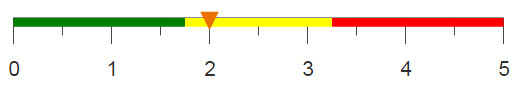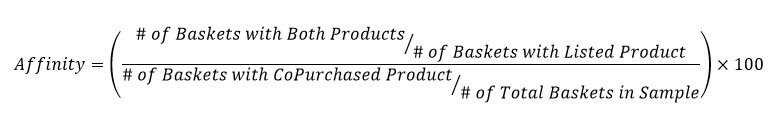# Performing an affinity analysis

You can determine the relationship of two items based on the amount of times the items were bought in the same transaction, the number of transactions that contained each item individually and the amount of transaction over all.

## Difficulty## Objective

You want to determine the affinity, or the relationship, between two items. Let's say, you are analyzing retail transactions and you want to know how likely it is for a certain item to be purchased with a different item. For example, hot dogs and hot dog buns have a high affinity. Or you are the owner if an ice cream shop and you want to know which flavor and which topping are most commonly ordered together.

## Solution

```<base table="pub.doc.retail.altseg.sales_detail_transid"/>
<sel value="between(trans_date;20150101;20160101)"/>
<sel value="xsales>0"/>
<note>Flag Primary Products</note>
<willbe name="sel" value="group=65"/>
<sel value="g_or(customer trans_date transid; ;sel)"/>
<willbe name="unique_trans"
value="g_last1(customer trans_date transid;;)"/>
<tabu breaks="sku" label="Tabulation">
<tcol source="transid" fun="ucnte" name="bskts_both"
label="# Baskets`with Both"/>
<tcol source="unique_trans" fun="sum" name="total_trans"/>
</tabu>
<willbe name="bskts_prod" value="g_sum(;;total_trans)"
label="# Baskets`with Product"/>
<willbe name="bskts_both_pct" value="bskts_both/bskts_prod*100"
label="% of Baskets`with Both" format="dec:2"/>
<note>Total Baskets of Co-Purchase Product</note>
<link table2="pub.doc.retail.altseg.sales_detail_transid" col="sku"
col2="sku">
<sel value="between(trans_date;20150101;20160101)"/>
<sel value="xsales>0"/>
<tabu breaks="sku" label="Tabulation">
<tcol source="transid" fun="ucnte" name="bskts_co"
label="# Baskets with`Co-Purchase Product"/>
</tabu>
</link>
<willbe name="linker" value="1"/>
<note>All Baskets in time/store range selection</note>
<link table2="pub.doc.retail.altseg.sales_detail_transid" col="linker"
col2="linker">
<sel value="between(trans_date;20150101;20160101)"/>
<sel value="xsales>0"/>
<tabu label="Tabulation">
<tcol source="transid" fun="ucnte" name="bskts_all"
label="Total # Baskets`in Sample"/>
</tabu>
<willbe name="linker" value="1"/>
</link>
<willbe name="bskt_aff" label="Affinity"
value="min(9999;int(bskts_both_pct/(bskts_co/bskts_all*100)*100))"/>
<sel value="bskts_both_pct>=.1"/>
<sel value="(bskts_both_pct<>100)&(bskts_co<>bskts_both)"/>
<sort col="bskt_aff" dir="down"/>
<sort col="sku" dir="up"/>
<note>Brings in SKU information - remove if not wanted</note>
<link table2="pub.doc.retail.altseg.products" col="sku" col2="sku"/>
<col name="dept" fixed="1"/>
<col name="group" fixed="1"/>
<colord cols="dept,group,sku,description,bskts_prod,bskts_co,
bskts_both,bskts_all,bskts_both_pct,bskt_aff"/>```

## Discussion

Determining the relationship between items is very useful, especially in retail. If you can figure out what items are commonly bought together you can use these insights to make more effective decisions. This recipe uses demo retail sales data to determine the relationship between an item bought within group 65 to every other item bought in that basket. Below is the formula used to calculate affinity and the basis for our actions in this recipe.The solution first flags every row that contains an item from group 65 and then, using g_or(G;S;X), selects all baskets that contain at least one of these items. A tabulation is completed by breaking on the item SKU to determine the number of unique transactions and the number of baskets that contain both the current item and an item from group 65. By calculating the sum of unique transactions we can then determine how many total baskets there were that contained an item from group 65. Thus, the percentage of baskets containing both items can then be obtained.

From here, we need to link in additional tables. Within each link, we can perform additional calculations. These include tabulations to determine the total number of baskets in our sample and the number of baskets that contain each additional item purchased with the item in group 65. A final column uses all of our computed information to determine the affinity score for each item pair.

## Further reading

If you would like to learn more about the functions and operations discussed in this recipe, click on the links below:

g_or(G;S;X)